Next: Steady State Flow under Up: Super- and Subsonic Flow Previous: Super- and Subsonic Flow   Contents

## Flow in the Laval Nozzle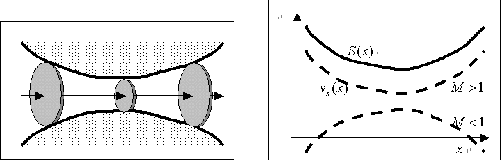Consider a tube whose cross-section,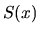, changes gradually, which is called Laval nozzle. Assuming the flow is steady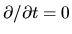and is essentially one-dimensional, the continuity equation (2.1) is rewritten as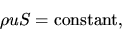(2.85)

or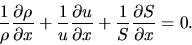(2.86)

Equation of motion (2.2) becomes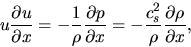(2.87)

where we used the relationship of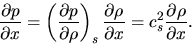(2.88)

When the flow is isothermal, use the isothermal sound speed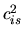instead of the adiabatic one. From equations (2.86) and (2.87), we obtain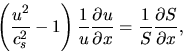(2.89)

where the factor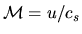is called the Mach number. For supersonic flow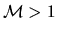, while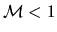for subsonic flow.

In the supersonic regime, the factor in the parenthesis of the lhs of equation (2.88) is positive. This leads to the fact that the velocity increases (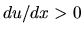) as long as the cross-section increases (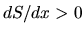). On the other hand, in the subsonic regime, the velocity decreases (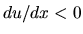) while the cross-section increases (). See right panel of 2.5.

If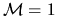at the point of minimum cross-section (throat), two curves forandhave an intersection. In this case, gas can be accelerated through the Laval nozzle. First, a subsonic flow is accelerated to the sonic speed at the throat of the nozzle. After passing the throat, the gas follows the path of a supersonic flow, where the velocity is accelerated as long as the cross-section increases.Next: Steady State Flow under Up: Super- and Subsonic Flow Previous: Super- and Subsonic Flow   Contents
Kohji Tomisaka 2007-07-08